## Artigo

•Citado por SciELO
•Acessos

•Similares em SciELO

## versão impressa ISSN 1665-2738

### Rev. Mex. Ing. Quím vol.10 no.2 Ciudad de México Ago. 2011

Simulación y control

Differential algebraic estimator for the monitoring of a class of partially known bioreactor models

Estimador algebraico diferencial para el monitoreo de una clase de biorreactores con modelos parcialmente conocidos

J. L. Mata–Machuca1, R. Martínez–Guerra1 and R. Aguilar–López2*

1 Departamento de Control Automático–CINVESTAV IPN.

2 Departamento de Biotecnología y Bioingeniería CINVESTAV–IPN . Av. Instituto Politécnico Nacional No. 2508, San Pedro Zacatenco, México, D.F. C.P 07360. *Corresponding author. E–mail: raguilar@cinvestav.mx Tel. + 52 55 5747 3800

Accepted 13 of May 2011.

Abstract

The problem of monitoring in a common class of partially know bioreactor models is a d d ressed. A reduced order observer namely differential algebraic estimator is proposed. The biomass is estimated by means of substrate concentration measure ments. The estimation methodology is based on a suitable change of variable which allows generating artificial variables to infer the remaining mass concentrations constructing a differential–algebraic structure. The proposed methodology is applied to a class of Haldane unstructured kinetic model with success. Stability analysis in a Lyapunov sense for the estimation error is performed. Some remarks about the convergence characteristics of the proposed estimator are given and numerical simulations show its satisfactory performance. Finally, for comparison purposes, a high gain observer is presented: the convergence is possible only when the model is perfectly known.

Keywords: differential–algebraic estimator, state variable estimation, continuous bioreactor, Haldane kinetics.

Resumen

En el presente trabajo se considera el problema del monitoreo de una clase de biorreactores con modelos parcialmente conocidos. Se propone un tipo de observador de orden reducido denominado estimador diferencial algebraico. La metodología de estimación se basa en un cambio de variables que permite generar variables artificiales para inferir las concentraciones no medibles. La metodología propuesta es aplicada a un modelo cinético no estructurado de Haldane con éxito. Se efectúa un análisis de Lyapunov para demostrar la estabilidad de la metodología considerada. Algunos comentarios sobre las características de la convergencia del estimador son proporcionados y simulaciones numéricas muestran un desempeño satisfactorio. Finalmente, con propósitos de comparación, un observador de alta ganancia se presenta en donde su convergencia se garantiza solo cuando se conoce perfectamente el modelo del sistema bajo estudio.

Palabras clave: estimador algebraico–diferencial, estimación de variables de estado, biorreactor continuo, cinética de Haldane.

1 Introduction

Operating a bioreactor is not a simple task, as during a bioreacting process, variables such as concentrations are generally determined by off–line laboratory analysis, making this set of variables of limited use for control purposes and on–line monitoring. However, these variables can be on–line estimated using soft sensors.

Over the last few years, the importance of on–line monitoring of biotechnological processes has increased. A first step to efficient bioreactor operation is the adequate implementation of online measurements of essential variables such as substrate and biomass concentrations. Advantages of continuous monitoring of key variables include gaining knowledge about the state of the process and the possibility of detecting and isolating abnormal process developments at early stages. This reduces process costs, contributes to process safety and helps in trouble–shooting and process accommodation. The main problem in fermentation monitoring and control is the fact that process variables usually cannot be measured on–line. Monitoring and controlling these processes can therefore be difficult because only indirect measurements are available online, while calculated values may be rather uncertain. This can be due to uncertainty with respect to the equations used, measurement errors or both. For automatic control this may have serious consequences, especially as the actual variables of interest often cannot be directly controlled and related variables are controlled instead. In fermentation processes, on–line and offline measurements are the main source of information about the state of the process. In combination with model–based calculations, they are used to produce estimations for monitoring purposes as well as for automatic and manual process control (Bastin and Dochain, 1990), (Masoud, 1997).

Observation schemes are widely used for reconstructing states of dynamical systems (Aguilar–López et al., 2006). Most of the contributions are related to asymptotic observers for monitoring, fault detections and control issues whereas the real necessities of industrial plants are related to a fast response of the monitoring and regulation methodologies.

Special attention was given to filtering techniques, namely extended Kalman filter, adaptive observers, and artificial neural networks (ANN), (Dávila and Fridman, 2005), (Hu and Wang, 2002), (Levant, 2001), however for these techniques the right tuning of the estimators gains is difficult. It is shown that software based state estimation is a powerful technique that can be successfully used to enhance automatic control performance of biological systems as well as in system monitoring and on–line optimization.

In this paper we consider the growth rate partially known. Following this idea, the necessity to adapt an observation scheme to the available knowledge of the growth rate immediately arises. The main contribution in this work is to show a state estimator which is a simplified version of the methodology given by (Lemesle and Gouzé, 2005) where a simple linear change of variable given in a natural manner allows to develop a differential–algebraic state estimator. Results show an adequate performance of the considered methodology. The technique is not the same as (Alvarez–Ramirez et al, 1999) since we do not have derivators. The proposed estimation methodology is applied to a kind of unstructured kinetic model: the Haldane model, which is considered for biological process with substrate inhibition. The above mentioned kinetic model is applied to a class of continuous stirred bioreactors.

In what follows, the statement of the problem is presented; an observability condition is given in the differential–algebraic setting. In section 3, the bounded error estimator is designed. Section 4 shows a high gain observer as a comparison with the proposed methodology. Finally, we give some concluding remarks.

2 Problem statement

2.1 The model

Consider the following nonlinear system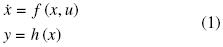where, xRn, uRm, m < n, yRp.

Let us recall the classical observer definition. An observer for system (1) is a dynamical system=(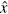, u, y), whose task is state estimation. Usually is required at least that ||x||0 as t∞. Although in some cases, exponentially convergence is also required (Gauthier et al., 1992).

Definition 1: an estimator is said to be bounded if the estimation error (||x||) belongs to an open ball with radius proportional to some value that depends on its estimation error.

In all paper, we will consider a class of bioreactor model. The simplified Haldane model taken from (Vargas et al., 2000), is described bywhere μ(S) = μmaxS / (δ + S + S2/φ) is the specific growth rate and μmax is the maximum growth rate.

We assume that μ(S) is partially known, which is common in biology (Gouzé and Lemesle, 2001). Generally, μ(S) is between two bounds meaning that we know a function(S) such that |μ(S) –(S)| < a, where aR+, and μ(0) =(0) = 0. We introduce an important lemma about lower bounded properties of μ(S).

Lema 1 (Hadj–Sadok, 1999): there exists a constant εR, such that S (0) > ε implies S(t) > ε for all t. Thus, for any smooth function μ(S), μ (S (t)) > μ (ε) for all t.

From lemma 1, we could always choose ε such that μ (S (t)) >(ε) = r, where rR+.

The state variables S, X are the substrate and biomass concentrations, respectively, D = q/V is the dilution rate with V the volume of the bioreactor and q the constant flow passing through the bioreactor, Sin is the input substrate concentration, YS/X is the corresponding yield coefficient. Let us notice that the inputs D = u and Sin are fixed. Moreover, we assume that the measured output is,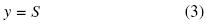2.2 Algebraic Observability Condition (AOC)

Before proposing the bounded error estimator, a definition concerning on algebraic observability condition is given, for more details see (Diop and Martínez–Guerra, 2001).

Definition 2: consider the system described by (1), where x = ( x1 x2 ... xn )T. A state xi, i = {1, 2, ... ,n}, is said to be algebraically observable with respect to {u,y} if it satisfies a differential polynomial in terms of u, y and some of their time derivatives, i.e., P(xi, u,, ..., y,,..) = 0, i = {1, 2, ... ,n}.

Replacing y = S into Eq. (2a), the algebraic observability condition for Haldane model is calculated as follows,From Eq. (4), it is clear that the state variable X satisfies the AOC thus, X is algebraically observable.

3 Bounded error estimator

3.1 Estimator design

In what follows, the corresponding estimated concentration is denoted by ^, and we assume that S is measured exactly, i.e., S =. Then, we only reconstruct the biomass variable X.

Consider the Haldane's model given by system (2), and make the change of variablewhere kR is fixed.

The dynamics of z isProposition 1: if we choose the estimator's gain such that YS/X < k1 + D/kd and |μ(S)(S)| < a, aR+. Then, the system (7) is a bounded error estimator of (6).For the proof, define the estimation error,Then, using eqs. (6) and (7) the estimation error dynamic is obtained as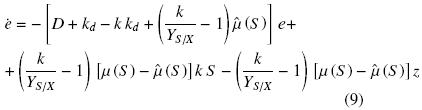To analyze the stability of Eq. (9) we consider the following Lyapunov function candidateThe time derivative of Eq. (10) isReplacing (9) into (11) yields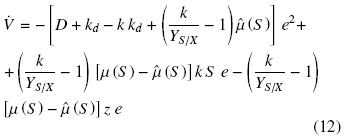Equation (12) is written alternatively asNow, from lemma 1 and taking into account that YS/X < k ≤ 1 + Dkd and |μ(S)(S)| < a, and X is bounded, Eq. (13) leads to,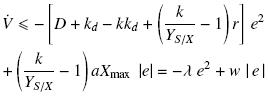where,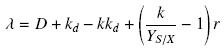and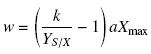The right–hand side of the foregoing inequality is not negative since near the origin, the positive linear term w |e| dominates the negative quadratic term –λe2. However,is negative outside the set {|e| = ≤ w/λ}. Let c, ε be some upper bounds for V(e). With c > w2 / 2λ2, solutions starting in the set {V(e)≤ c} will remain therein for all time becauseis negative on the boundary V = c. Hence, the solutions of Eq. (9) are uniformly bounded (Khalil, 2002). Moreover, if (w2 / 2λ2) < ε < c, thenwill be negative in the set {ε≤ V ≤ c}, which shows that, in this set V will decrease monotonically until the solutions enters the set {V ≤ ε}. From that time on, the solution cannot leave the set {V ≤ ε}sinceis negative on the boundary V = ε. According to (Khalil, 2002), the solution is uniformly ultimately bounded with the ultimate bound |e| V2e. For instance, defining c and ε as follows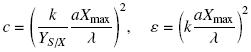the ultimate bound is, |e|.

Corollary 1: if the growth rate is perfectly known, i.e., μ (S) =(S), and we choose the estimator's gain such that YS/X < k ≤ 1 + D/kd. Then, the system (14) is an asymptotic estimator of (6).Indeed, the dynamics of the error in this case is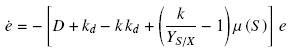and the corresponding time derivative of Lyapunov function candidate (10) isMoreover, X can be reconstructed considering3.2 Numerical simulations

For all simulations in this paper we take Sin = 50, D = 0.1, YS/X = 0.9, kd = 0.01 and the initial conditions S (0) = 60, X(0) = 40,(0) = 30,(0) = 90, with appropriate units, these values are taken from Vargas et al, (2000). The estimator's gain is k = 1. The growth rates are chosen asandwhen the model is well known for the asymptotic estimator and when the model is partially known for the bounded error estimator, respectively. The simulations results were carried out with the help of Matlab 7.1 Software with Simulink 6.3 as the toolbox.

The performance index of the corresponding estimation process is calculated as (Martínez–Guerra et al., 2000)where e(t) is the corresponding state estimation error (the difference between the actual observed signal and its estimmate).

First, in Fig. 1 we show the simulation results for the bounded error estimator given by proposition 1, and the corresponding results for the asymptotic estimator given by corollary 1 (without any noise in the system output). Furthermore, in Fig. 2 is shown the effect of noise in the estimation process. A white noise is added in the measurement (σ= 0.1, ±10% around the current value of the measured output) this is considering the corres ponding measurement error of the corresponding sensor and/or experimental measurement technique. We can observe that the bounded error estimator is robust against noisy measurement. Finally, in Fig. 3 is illustrated the performance index given by (16) for the corresponding estimation process. It should be noted that the quadratic estimation error (performance index) is bounded on average and has a tendency to decrease.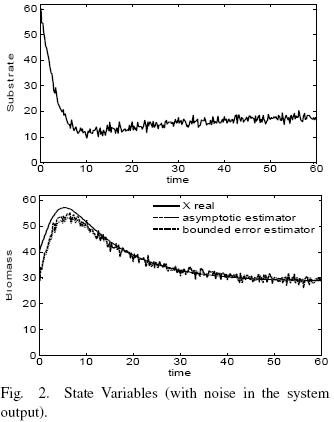4 A note on full–order observers: The high gain observer

4.1 Observer design

Consider that system (1) satisfies the AOC. In this case to estimate the state–space vector x, we can suggest a nonlinear high gain observer (Gauthier et ah, 1992), (Martínez–Guerra et al, 2000) with the following structure,where the observer's g ain matrix is given by,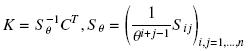and the positive parameter θ determines the desired convergence velocity. Moreover, Sθ > 0, Sθ =should be a solution of the algebraic equation,As shown by (Gauthier et ai, 1992), (Martínez Guerra and de Leon–Morales, 1996), under certain technical a assumptions (Lipschitz conditions for nonlinear functions under consideration) this nonlinear observer has an arbitrary exponential decay for any initial conditions. We obtain the following high order observer for the system (2) applying the observation scheme (17),4.2 Simulations

In the same way, we 8show two simulations: when the model is well known and when the model is partially known. The initial conditions for the observer are(0) = 40,(0) = 30, with appropriate units. The estimator's gain is θ = 2. The simulations results of high gain observer are presented in figs. 4 and 5. In Fig. 4, without any noise in the system output, when the model is perfectly known the rate of convergence is fast, on the other hand, when the model is partially known the observer does not reconstruct the state variables. In Fig. 5, we studied the effect of noise in the measurement (white noise with σ = 0.1, +5% around the current value of the measured output), we can see that the high gain observer is very sensitive to the noise in the system output. Fig. 6 shows the performance index. It should be noted that this observer only reconstruct the state variables when the model is well known.Conclusions

In this paper we ha ve present ed a boun ded error estimator for bioprocess with unstructured growth models. We have proven the stability of the corresponding estimation error in a Lyapunov sense. By means of a linear change of variable given in a natural manner and with some algebraic manipulations have been constructed the state estimator, which converges to the current states of the reference model given. We have demonstrated that the bounded error estimator under consideration provides good enough state–space estimates which were bounded on average, besides the proposed state estimator does not depend of a particular set of initial conditions or specific model structure. Moreover, we have constructed a high gain observer in which the convergence is fast only if the model is well known, but does not exists convergence if the model is partially known. Finally, we have presented some simulations to illustrate the effectiveness of the suggested approach, which shows some robustness properties against noisy measurements.

References

Aguilar–López, R., Martínez–Guerra, R., Mendoza–Camargo, J., and M. Neria–González (2006). Monitoring of and industrial wastewater plant employing finite–time convergence observer. Journal of Chemical Technology and Biotechnology 81, 851–857.         [ Links ]

Alvarez–Ramirez, J (1999). Robust PI stabilization t). of a class of continuously stirred–tank reactors. AIChE Journal 45, 1992–2000.         [ Links ]

Bastin, G. and D. Dochain(1990). On–line estimation and adaptative control of bioreactors 1. Elsevier, Amsterdam.         [ Links ]

Dávila, J., Fridman, L. and A. Levant (2005). Second order sliding–mode observer for mechanical systems. IEEE Transactions on Automatic Control 50, 1785–1789.         [ Links ]

Diop, S. and R. Martínez–Guerra (2001). An algebraic and data derivative information approach to nonlinear system diagnosis. Proceedings of the European Control Conference (ECC), Porto, Portugal, 2334–2339.         [ Links ]

Farza, M., Busawon, K. and H. Hammouri (1998). Simple nonlinear observers for online estimation of kinetics rates in bioreactors. Automatica 34, 301–318.         [ Links ]

Gauthier, J., Hammouri H. and S. Othman (1992). A simple observer for nonlinear systems. Applications to bioreactors. IEEE Transactions on Automatic Control 37, 875–880.         [ Links ]

Gouzé, J. and V. Lemesle (2001). A bounded error observer for a class of bioreactor models. Proceeding of the European Control Conference (ECC), Porto, Portugal.         [ Links ]

Hadj–Sadok, Z. (1999). Modélisation et estimation dans les bioréacteurs; prise en compte des incertudes: application au traitement de l'eau. PhD thesis. Nice–Sophia Antipolis University. Nice.         [ Links ]

Hu, S. and J. Wang (2002). Global asymptotic stability and global exponential stability of continuous time recurrent neural netwoks. IEEE Transactions on Automatic Control 47, 802–807.         [ Links ]

Keller, H (1987). Non–linear observer design by transformation into a generalized observer canonical form. International Journal of Control 46, 1915–1930.         [ Links ]

Khalil, H (2002). Nonlinear systems. Third edition. Prentice Hall, New Jersey.         [ Links ]

Lemesle, V. and J. Gouzé (2005). Hybrid bounded error observers for uncertain bioreactor models. Bioprocess & Biosystems Engineering 27, 311–318.         [ Links ]

Levant, A (2001). Universal single–input–single–output (SISO) sliding–mode controllers with finite–time convergence. IEEE Transactions on Automatic Control 46, 1447–1451.         [ Links ]

Luenberger, D (1979). Introduction to Dynamic Systems. Theory Models and Applications. Wiley, New York.         [ Links ]

Martínez–Guerra, R. and J. de Leon–Morales (1996). Nonlinear estimators: a differential algebraic approach. Journal of Mathematics and Computer Modelling 20, 125–132.         [ Links ]

Martínez–Guerra, R., Poznyak, A. and V. Díaz (2000). Robustness of high–gain observers for closed–loop nonlinear systems: theoretical study and robotics control application. International Journal of Systems Science 31, 1519–1529.         [ Links ]

Masoud, S (1997). Nonlinear state–observer design with application to reactors. Chemical Engineering Science 52, 387–404.         [ Links ]

Vargas, A., Soto, G., Moreno, J. and G. Buitrón (2000), Observer based time–optimal control of an aerobic SBR for chemical and petrochemical wastewater treatment. Water Science and Technology 42, 163–170.         [ Links ]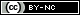Todo o conteúdo deste periódico, exceto onde está identificado, está licenciado sob uma Licença Creative Commons